Machine_Parts_Index
Power screw Page

Power Screw Strength Equations

Strength considerations for Power Screws

Introduction

The following notes are provided for general guidance.  In practice power screws are provided by specialist suppliers who provide technical literature which includes all the necessary data for selecting power screws from their range.   The notes below are general in nature and cannot provide detailed information about precise strength levels because there are limitations on the understanding of the stress levels in screws.   Calculations assume that loading is distributed along the whole length of the engaged screw.. In practice this is not the case and the loading is actually mainly taken by the first two threads. These may yield a little to distribute the load along the thread.

The stress levels are also effected by thread finish, clearance, shape,lubrication etc etc. The following factors need to be considered in calculating the strength of a power screw

 Bearing Strength Bending Strength Shear Strength Direct tensile/compressive strength Direct tensile/compressive strength Column strength

Symbols

Bearing Stress

The bearing stress results from the crushing force between the screw surface and the adjacent nut surface developed by lifting and supporting the load W.

σ B = W / ( π . dm. h.n )

Table of Safe Bearing Pressures

 Screw Mat Nut Mat Bearing Pressure Notes Steel Bronze 17-24 N/mm2 Low Speed Steel Bronze 11-17 N/mm2 3 m/min Steel Cast Iron 13-17 N/mm2 2,5m /min Steel Bronze 5-10 N/mm2 6-12 m/min Steel Cast Iron 7-7 N/mm2 6-12 m/min Steel Bronze 1- 2 N/mm2 15 m/min

Bending Stress

The maximum bending stress occurs at the root of the thread.  It is calculated by assuming the thread is a simple cantilever beam built in at the root.  The load is assumed to act at mid point on the thread.

The maximumum stress is provided by the bending moment relationship M/I = σ /(y) =e/R. that is σ = M.y/I

The section under bending has a length = π.dm.n
The width of the section at the thread root = b.
The Moment of Inertia at the root I = π.dm.n.b3 /12
The distance from the centroid the the most remote fibre ..y = b/2.
The Bending Moment M = W.h/2
The maximum bending stress is therefore..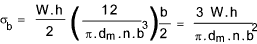For an ISO metric screw thread b = 3p /4 ... for a square single start thread b = p /2

Shear Stress

Both the nut and screw threads are subject to traverse shear stress resulting from the bending forces.  For a rectangular section the maximums shear stress occurs at the neutral axis and equals

Screw...τ = 3.W /2.A = 3.W /(2.π.d r.n.b )
Nut...τ = 3.W /2.A = 3.W /(2.π.d o.n.b )

Tensile /Compressive stresses

A loaded power screw is subject to a direct tensile or compressive load..  This is simply calculated as the load / tensile stress area.

The tensile stress area is generally provided in screw tables and is generally larger than that calculated by the root dia.  Using a stress area based on the root diameter may be used for conservative design studies. (A = πdr2/4)

The preferred stress area is actually based on the (pitch dia + root dia)/2.. For ISO metric screws this is illustrated at section Stress area -ISO 898

σ t or c = W /A...

A = π d t 2 / 4

d t = (dp + dr) /2

Combined stresses

Based on maximum shear stress theory...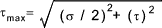The shear stress τ caused by torque on the screw =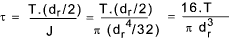The value of the combined stress is therefore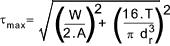This equation always applies when the screw is in tension.   When a screw is in compression and the length is greater than 8 time the root diameter then the buckling stress has to be considered..

Buckling stress..ref Simple Struts

When the screw is longer than 8 times the root diameter it must be considered a column.   Long columns with are dealt with using the Euler equation. Columns with slenderness ratios of less than 100 are considered as short columns.  The slenderness ratio is the length (between supports) / Least radius of gyration of the section.

For Machine tool design a variation on the Gordon-Rankine formula is used.   This is called the Ritter equation ..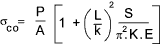For a column the maximum stress at the concave side of the column σ co should not exceed the design compressive strength of the screw material..

K = factor to allow the column end supports

 One end fixed and one end free K= 0,25 Both ends pin-connected K= 1 One end pin-connected and one end fixed K= 2 Both ends fixed K= 4

The above equation applies only the screws with purely axial loads.   When the load is eccentric from the screw centre line by distance e.  Then the following variation of the Ritter equation applies.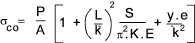Links to Power Screw Design Power Screw ...UTM Powerpoint Presentation (Ball screws) Power screw calculation ... Useful ABC calculator FAQ screw Jacks ...General information on power screws from Powerjacks DAN Notes -Screw Threads ...Extremely useful Notes
Machine_Parts_Index
Power screw Page